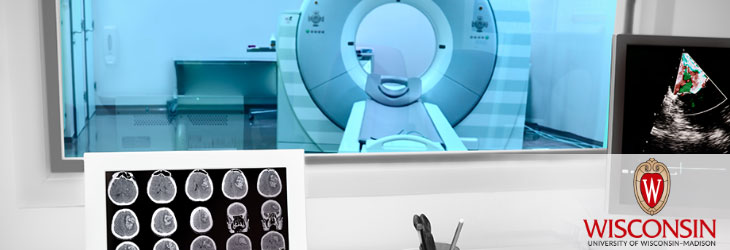Wisconsin Alumni Research Foundation

# WARF TECHNOLOGIES

Medical ImagingGeneralized Method For Mri Chemical Species Separation Using Arbitrary K-space Trajectories
WARF: P07261US

Inventors: Scott Reeder

The Invention
A method for producing images of a subject containing M spin species using a magnetic resonance imaging (MRI) system includes obtaining N k-space data matrices from N k-space data sets acquired with the MRI system using a pulse sequence with an individual associated echo time. The k-space data matrices each include corresponding data at the same plurality of k-space locations and time stamps are tracked for each k-space location. For each k-space location, a set of linear equations in k-space is solved. The set of linear equations relates corresponding data from the N k-space data matrices, echo times and time stamps to desired calculated k-space data. Calculated data in k-space which is corrected for chemical shift is produced corresponding to each k-space location and aggregated to obtain a k-space calculated data set. The k-space calculated data set is transformed to image space to obtain a corresponding image.